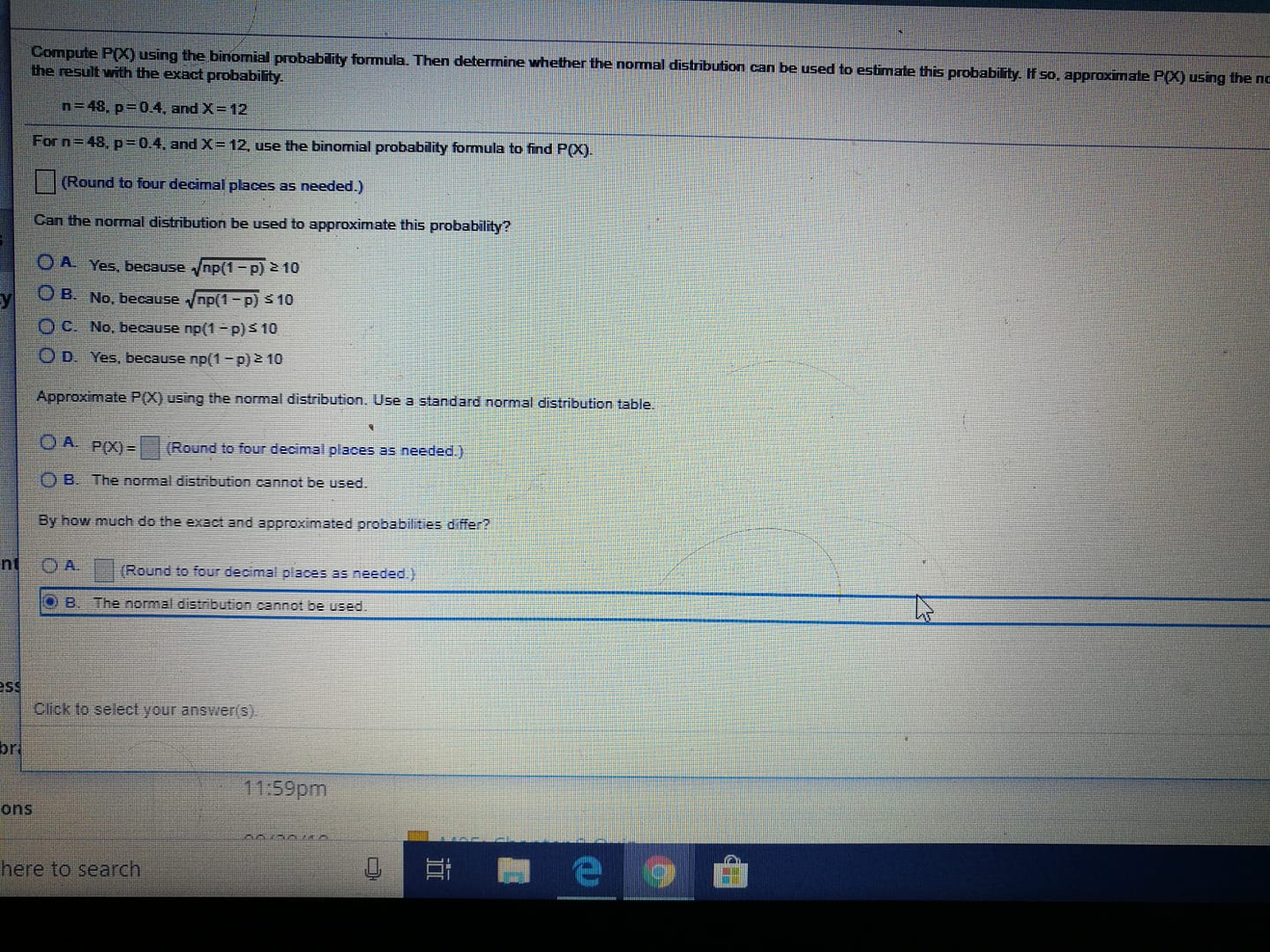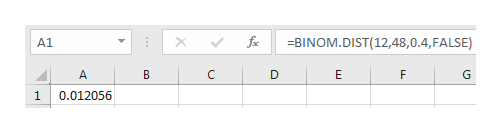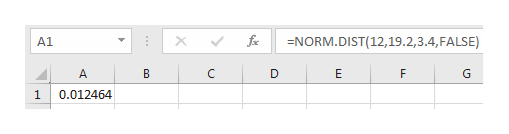# Compute P) using the binomial probability formula. Then deternine whether the normal distribution can be used to estimate this probability. If so, approximate P(X) using the ncthe result with the exact probabilityn 48, p 0.4, and X12For n= 48, p=0.4, and X= 12, use the binomial probability formula to find P(X).(Round to four decimal places as needed.)Can the normal distribution be used to approximate this probability?OAYes, because /np(1-p) 2 10O B. No, because np(1-p) \$ 10O C. No, because np(1-p)s 10O D. Yes, because np(1-p) 2 10Approximate P(X) using the normal distribution. Use a standard normal distribution tableOA PX)=(Round to four decimal places as needed.)B. The normal distribution cannot be used.By how much do the exact and approximated probabilities differ?ntO A.(Round to four decimal places as needed)O B. The normal distribution cannot be usedssClick to select your answer(s).r11:59pmonshere to search

Questionhelp_outlineImage TranscriptioncloseCompute P) using the binomial probability formula. Then deternine whether the normal distribution can be used to estimate this probability. If so, approximate P(X) using the nc the result with the exact probability n 48, p 0.4, and X 12 For n= 48, p=0.4, and X= 12, use the binomial probability formula to find P(X). (Round to four decimal places as needed.) Can the normal distribution be used to approximate this probability? OA Yes, because /np(1-p) 2 10 O B. No, because np(1-p) \$ 10 O C. No, because np(1-p)s 10 O D. Yes, because np(1-p) 2 10 Approximate P(X) using the normal distribution. Use a standard normal distribution table OA PX)= (Round to four decimal places as needed.) B. The normal distribution cannot be used. By how much do the exact and approximated probabilities differ? nt O A. (Round to four decimal places as needed) O B. The normal distribution cannot be used ss Click to select your answer(s). r 11:59pm ons here to search fullscreen
check_circleExpert Solution
Step 1

It is given that n=48, p=0.4.

The required binomial probability, P(X=12) can be obtained by entering the formula ‘=BINOM.DIST(12,48,0.4,FALSE)’ in EXCEL software.help_outlineImage TranscriptioncloseBINOM.DIST(12,48,0.4, FALS E) A1 A B C E F G 1 0.012056 O X fullscreen
Step 2

The normal distribution can be approximate this probability because,

Here, np(1–p) =11.52 which is greater than or equal to 10.

Therefore, Option D is correct.

Now consider the mean and standard deviation of the normal distribution.

Step 3

Therefore, the random variable X follows normal distribution with mean 19.2 and standard deviation 3.4.

The required normal probability, P(X=12) can be obta...help_outlineImage Transcriptionclose=NORM.DIST(12, 19.2, 3.4, FALSE) A1 A B C E F G 1 0.012464 X fullscreen

### Want to see the full answer?

See Solution

#### Want to see this answer and more?

Solutions are written by subject experts who are available 24/7. Questions are typically answered within 1 hour*

See Solution
*Response times may vary by subject and question
Tagged in

### Statistics